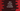# Java program to convert a string to boolean### Java program to convert a string to boolean using valueOf() :

In this example, we will learn how to convert a string to boolean value. For that, we will use one static method valueOf(String) of the Boolean class. This class is defined as below :

``````public static Boolean valueOf(String s) {
return parseBoolean(s) ? TRUE : FALSE;
}``````

So, it calls parseBoolean() method. It is defined as below :

``````public static boolean parseBoolean(String s) {
return ((s != null) && s.equalsIgnoreCase("true"));
}``````

So, if we will put input as true , it will return boolean true and else return false.

### Java program :

``````import java.util.Scanner;

public class Main {

public static void main(String[] args) throws java.lang.Exception {
//1
Scanner scanner = new Scanner(System.in);

//2
String str = scanner.next();

//3
Boolean booleanValue = Boolean.valueOf(str);

//4
System.out.print("Boolean value is "+booleanValue);
}

}``````

### Sample Output :

``````TruE
Boolean value is true

true
Boolean value is true

truE
Boolean value is true

false
Boolean value is false

one
Boolean value is false``````